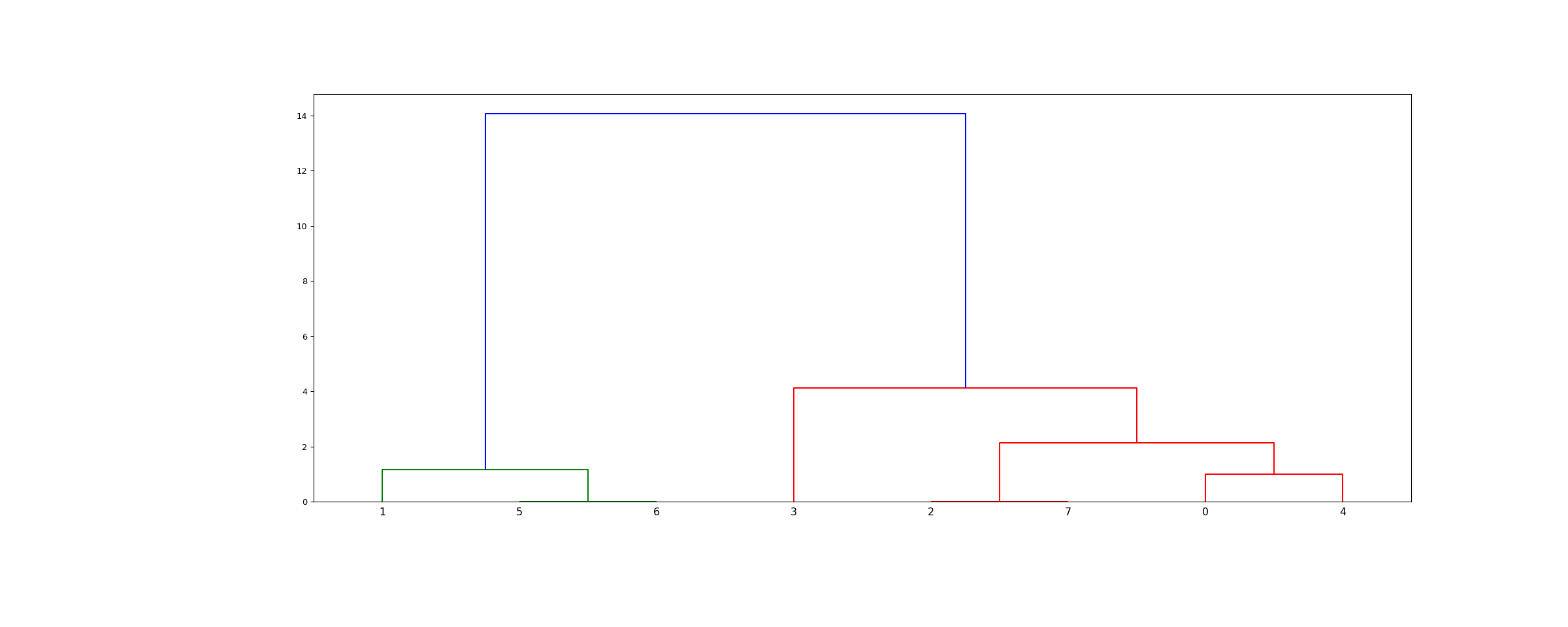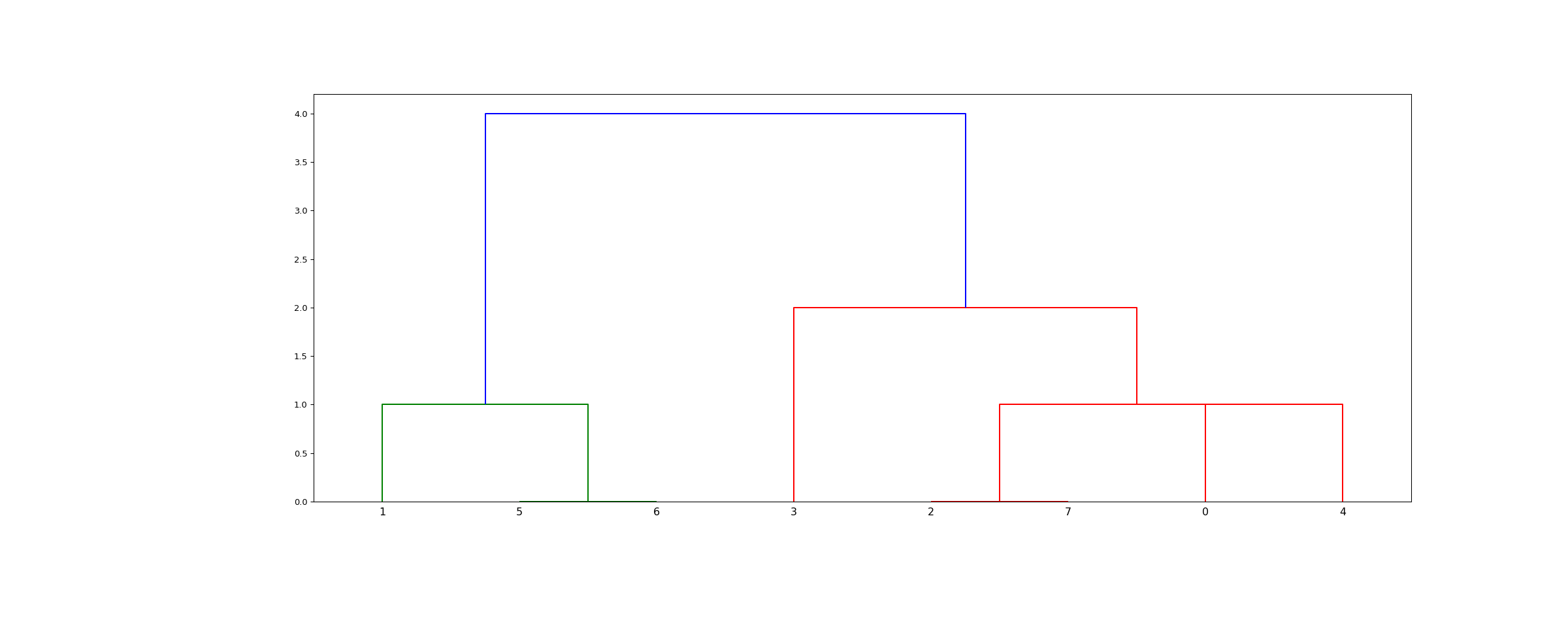scipy.cluster.hierarchy.linkage(y, method='single', metric='euclidean', optimal_ordering=False)[source]

Perform hierarchical/agglomerative clustering.

The input y may be either a 1d condensed distance matrix or a 2d array of observation vectors.

If y is a 1d condensed distance matrix, then y must be a $$\binom{n}{2}$$ sized vector where n is the number of original observations paired in the distance matrix. The behavior of this function is very similar to the MATLAB linkage function.

A $$(n-1)$$ by 4 matrix Z is returned. At the $$i$$-th iteration, clusters with indices Z[i, 0] and Z[i, 1] are combined to form cluster $$n + i$$. A cluster with an index less than $$n$$ corresponds to one of the $$n$$ original observations. The distance between clusters Z[i, 0] and Z[i, 1] is given by Z[i, 2]. The fourth value Z[i, 3] represents the number of original observations in the newly formed cluster.

The following linkage methods are used to compute the distance $$d(s, t)$$ between two clusters $$s$$ and $$t$$. The algorithm begins with a forest of clusters that have yet to be used in the hierarchy being formed. When two clusters $$s$$ and $$t$$ from this forest are combined into a single cluster $$u$$, $$s$$ and $$t$$ are removed from the forest, and $$u$$ is added to the forest. When only one cluster remains in the forest, the algorithm stops, and this cluster becomes the root.

A distance matrix is maintained at each iteration. The d[i,j] entry corresponds to the distance between cluster $$i$$ and $$j$$ in the original forest.

At each iteration, the algorithm must update the distance matrix to reflect the distance of the newly formed cluster u with the remaining clusters in the forest.

Suppose there are $$|u|$$ original observations $$u, \ldots, u[|u|-1]$$ in cluster $$u$$ and $$|v|$$ original objects $$v, \ldots, v[|v|-1]$$ in cluster $$v$$. Recall $$s$$ and $$t$$ are combined to form cluster $$u$$. Let $$v$$ be any remaining cluster in the forest that is not $$u$$.

The following are methods for calculating the distance between the newly formed cluster $$u$$ and each $$v$$.

• method=’single’ assigns

$d(u,v) = \min(dist(u[i],v[j]))$

for all points $$i$$ in cluster $$u$$ and $$j$$ in cluster $$v$$. This is also known as the Nearest Point Algorithm.

• method=’complete’ assigns

$d(u, v) = \max(dist(u[i],v[j]))$

for all points $$i$$ in cluster u and $$j$$ in cluster $$v$$. This is also known by the Farthest Point Algorithm or Voor Hees Algorithm.

• method=’average’ assigns

$d(u,v) = \sum_{ij} \frac{d(u[i], v[j])} {(|u|*|v|)}$

for all points $$i$$ and $$j$$ where $$|u|$$ and $$|v|$$ are the cardinalities of clusters $$u$$ and $$v$$, respectively. This is also called the UPGMA algorithm.

• method=’weighted’ assigns

$d(u,v) = (dist(s,v) + dist(t,v))/2$

where cluster u was formed with cluster s and t and v is a remaining cluster in the forest. (also called WPGMA)

• method=’centroid’ assigns

$dist(s,t) = ||c_s-c_t||_2$

where $$c_s$$ and $$c_t$$ are the centroids of clusters $$s$$ and $$t$$, respectively. When two clusters $$s$$ and $$t$$ are combined into a new cluster $$u$$, the new centroid is computed over all the original objects in clusters $$s$$ and $$t$$. The distance then becomes the Euclidean distance between the centroid of $$u$$ and the centroid of a remaining cluster $$v$$ in the forest. This is also known as the UPGMC algorithm.

• method=’median’ assigns $$d(s,t)$$ like the centroid method. When two clusters $$s$$ and $$t$$ are combined into a new cluster $$u$$, the average of centroids s and t give the new centroid $$u$$. This is also known as the WPGMC algorithm.

• method=’ward’ uses the Ward variance minimization algorithm. The new entry $$d(u,v)$$ is computed as follows,

$d(u,v) = \sqrt{\frac{|v|+|s|} {T}d(v,s)^2 + \frac{|v|+|t|} {T}d(v,t)^2 - \frac{|v|} {T}d(s,t)^2}$

where $$u$$ is the newly joined cluster consisting of clusters $$s$$ and $$t$$, $$v$$ is an unused cluster in the forest, $$T=|v|+|s|+|t|$$, and $$|*|$$ is the cardinality of its argument. This is also known as the incremental algorithm.

Warning: When the minimum distance pair in the forest is chosen, there may be two or more pairs with the same minimum distance. This implementation may choose a different minimum than the MATLAB version.

Parameters: y : ndarray A condensed distance matrix. A condensed distance matrix is a flat array containing the upper triangular of the distance matrix. This is the form that pdist returns. Alternatively, a collection of $$m$$ observation vectors in $$n$$ dimensions may be passed as an $$m$$ by $$n$$ array. All elements of the condensed distance matrix must be finite, i.e. no NaNs or infs. method : str, optional The linkage algorithm to use. See the Linkage Methods section below for full descriptions. metric : str or function, optional The distance metric to use in the case that y is a collection of observation vectors; ignored otherwise. See the pdist function for a list of valid distance metrics. A custom distance function can also be used. optimal_ordering : bool, optional If True, the linkage matrix will be reordered so that the distance between successive leaves is minimal. This results in a more intuitive tree structure when the data are visualized. defaults to False, because this algorithm can be slow, particularly on large datasets . See also the optimal_leaf_ordering function. New in version 1.0.0. Z : ndarray The hierarchical clustering encoded as a linkage matrix.

scipy.spatial.distance.pdist
pairwise distance metrics

Notes

1. For method ‘single’ an optimized algorithm based on minimum spanning tree is implemented. It has time complexity $$O(n^2)$$. For methods ‘complete’, ‘average’, ‘weighted’ and ‘ward’ an algorithm called nearest-neighbors chain is implemented. It also has time complexity $$O(n^2)$$. For other methods a naive algorithm is implemented with $$O(n^3)$$ time complexity. All algorithms use $$O(n^2)$$ memory. Refer to  for details about the algorithms.
2. Methods ‘centroid’, ‘median’ and ‘ward’ are correctly defined only if Euclidean pairwise metric is used. If y is passed as precomputed pairwise distances, then it is a user responsibility to assure that these distances are in fact Euclidean, otherwise the produced result will be incorrect.

References

  (1, 2) Daniel Mullner, “Modern hierarchical, agglomerative clustering algorithms”, arXiv:1109.2378v1.
  (1, 2) Ziv Bar-Joseph, David K. Gifford, Tommi S. Jaakkola, “Fast optimal leaf ordering for hierarchical clustering”, 2001. Bioinformatics DOI:10.1093/bioinformatics/17.suppl_1.S22

Examples

>>> from scipy.cluster.hierarchy import dendrogram, linkage
>>> from matplotlib import pyplot as plt
>>> X = [[i] for i in [2, 8, 0, 4, 1, 9, 9, 0]]

>>> Z = linkage(X, 'ward')
>>> fig = plt.figure(figsize=(25, 10))
>>> dn = dendrogram(Z)

>>> Z = linkage(X, 'single')
>>> fig = plt.figure(figsize=(25, 10))
>>> dn = dendrogram(Z)
>>> plt.show()